# 1 Introduction and Background (Tuesday, January 11)

References: .

Idea: study representation by studying associated geometric objects, and use homological methods to bridge the two. The representation theory side will mostly be rings/modules, and the geometric side will involve algebraic geometry and commutative algebra. Throughout the course, all rings will be unital and all actions on the left.

Recall the definition of a left $$R{\hbox{-}}$$module. Some examples:

• $$k\in \mathsf{Field}\implies {\mathsf{k}{\hbox{-}}\mathsf{Mod}} = { \mathsf{Vect} }_k$$
• $$R={\mathbb{Z}}\implies {\mathsf{{\mathbb{Z}}}{\hbox{-}}\mathsf{Mod}} = {\mathsf{Ab}}{\mathsf{Grp}}$$.
• $$A\in{\mathsf{Alg}}_{/ {k}}$$, which is a ring $$(A, +, \cdot)$$ where $$(A, +, .)$$ (using scalar multiplication) is a vector space.
• E.g. $$\operatorname{Mat}(n\times n, {\mathbb{C}})$$.
• E.g. for $$G$$ a finite group, the group algebra $$kG$$ for $$k\in \mathsf{Field}$$.
• E.g. $$U({\mathfrak{g}})$$ for $${\mathfrak{g}}\in \mathsf{Lie}{\mathsf{Alg}}$$ or a super algebra.

Connecting this to representation theory: for $$A\in {\mathsf{Alg}}_{/ {k}}$$ and $$M\in {\mathsf{A}{\hbox{-}}\mathsf{Mod}}$$, a representation of $$A$$ is a morphism of algebras $$A \xrightarrow{\rho} {\mathfrak{gl}}_n(k)$$, the algebra of all $$n\times n$$ matrices (not necessarily invertible). Note that for groups, one instead asks for maps $$kG\to \operatorname{GL}_n$$, the invertible matrices. There is a correspondence between $${\mathsf{A}{\hbox{-}}\mathsf{Mod}} \rightleftharpoons{\mathsf{Rep}}(A)$$: given $$M$$, one can define the action as \begin{align*} \rho: A &\to \mathop{\mathrm{End}}_k(M) \\ \rho(a)(m) &= a.m .\end{align*}

Recall the definitions of:

• Morphisms of $$R{\hbox{-}}$$modules: $$f(r.m_1 + m_2) = r.f(m_1) + f(m_2)$$
• Submodules: $$N\leq M \iff r.n \in N$$ and $$N$$ is closed under $$+$$.
• Quotient modules: $$M/N = \left\{{m + N}\right\}$$.
• The fundamental homomorphism theorem: for $$M \xrightarrow{f} N$$, there is an induced $$\psi: M/\ker f\to N$$ where $$M/\ker f\cong \operatorname{im}f$$.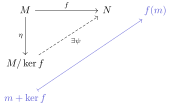• The fundamental SES \begin{align*} 0\to \ker f \xhookrightarrow{g} M \xrightarrow{f} \operatorname{im}f \to 0 ,\end{align*} where one generally needs $$\operatorname{im}g = \ker f$$ for exactness.
• More generally, need monomorphisms, epimorphisms.

Some examples:

• $$f:{\mathbb{Z}}\to {\mathbb{Z}}$$ where $$f(m) \mathrel{\vcenter{:}}= 4m$$ yields $$0\to {\mathbb{Z}}\xrightarrow{f} {\mathbb{Z}}\to {\mathbb{Z}}/4\to 0$$ in $${\mathsf{{\mathbb{Z}}}{\hbox{-}}\mathsf{Mod}}$$.
• In $${\mathsf{{\mathbb{C}}}{\hbox{-}}\mathsf{Mod}}$$, one can take $$0\to {\mathbb{C}}\xrightarrow{\Delta: x\mapsto (x, x)} {\mathbb{C}}{ {}^{ \scriptscriptstyle\times^{2} } } \to {\mathbb{C}}\to 0$$.

Direct sums, products, and indecomposables. Let $$I$$ be an index set and $$\left\{{M_k}\right\}_{k\in I}$$ $$R{\hbox{-}}$$modules to define the direct product $$\prod_{k\in I} M_k \mathrel{\vcenter{:}}=\left\{{(m_k)_{k\in I} {~\mathrel{\Big\vert}~}m_k\in M_k }\right\}$$, the set of all ordered sequences of elements from the $$M_k$$, with addition defined pointwise. For the direct sum $$\bigoplus _{k\in I} M_k$$ to be those sequences with only finitely many nonzero components. For internal direct sums, if $$M = M_1 + M_2$$ then $$M \cong M_1 \oplus M_2$$ iff $$M \cap M_2 = 0$$. An irreducible representation is a simple $$R{\hbox{-}}$$module, and an indecomposable representation is an indecomposable $$R{\hbox{-}}$$module. An $$R{\hbox{-}}$$module is simple iff its only submodules are $$0, M$$, and indecomposable iff $$M \not\cong M_1 \oplus M_2$$ for any $$M_i\not\cong M$$. Note that simple $$\implies$$ indecomposable.

Note: is it possible for $$M \cong M \oplus M$$?

Some examples:

• Simple objects in $${\mathsf{k}{\hbox{-}}\mathsf{Mod}}$$ are isomorphic to $$k$$, and indecomposables are also isomorphic to $$k$$ if we restrict to finite dimensional modules.

• Simple objects in $${\mathsf{{\mathbb{Z}}}{\hbox{-}}\mathsf{Mod}}$$ are cyclic groups of prime order, $$C_p$$. Indecomposables are $${\mathbb{Z}}, C_{p^k}$$, using the classification theorem to rule out composites.

• For $$A\in {\mathsf{Alg}}^{\mathrm{fd}}_{/ {k}}$$, the simple objects in $${\mathsf{A}{\hbox{-}}\mathsf{Mod}}$$ are hard to determine in general. The same goes for indecomposables, and is undecidable in many cases (equivalent to the word problem in finite groups).

See finite, tame, and wild representation types.

Toward homological algebra: free and projective modules. An $$R{\hbox{-}}$$module $$M$$ is free iff $$M\cong \bigoplus_{i\in I} R_i$$ for some indexing set where $$R_i \cong R$$ as a left $$R{\hbox{-}}$$module. Equivalently, $$M$$ has a linearly independent spanning set, or there exists an $$X$$ and a unique $$\phi$$ such that the following diagram commutes:Every $$M\in{\mathsf{R}{\hbox{-}}\mathsf{Mod}}$$ is the image of a free $$R{\hbox{-}}$$module: let $$X\mathrel{\vcenter{:}}=\left\{{m_i}\right\}_{i\in I}$$ generate $$M$$, so $$X\hookrightarrow M$$ by inclusion. Define $$X \to \bigoplus \bigoplus_{i\in I} R_i$$ sending $$m_i \to (0,\cdots, 1, \cdots, 0)$$ with a 1 in the $$i$$th position, then since $$X$$ is a generating set this will lift to a surjection $$\bigoplus _i R_i\to M$$. We can use this to define a free resolution: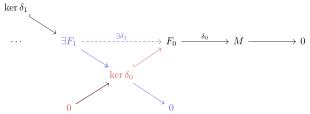Let $$A\in {\mathsf{Alg}}^{\mathrm{fd}}_{/ {k}}$$ and $$F \cong \bigoplus A$$ be free, and suppose $$e\in A$$ is idempotent, so $$e^2 = e$$ – these are useful because they can split algebras up. There is a Pierce decomposition of $$1$$ given by $$1 = e + (1-e)$$. Noting that $$1-e$$ is also idempotent, there is a decomposition $$A \cong Ae \oplus A(1-e)$$. Since $$Ae$$ is direct summand of $$A$$ which is free, this yields a way to construct projective modules.

# 2 Thursday, January 13

Last time:

• $$R{\hbox{-}}$$modules and their morphisms
• Free resolutions $$F \twoheadrightarrow R$$.

Today: projective modules and their resolutions.

See Krull-Schmidt theorem.

Recall the definition of projective modules $$P$$ and injective modules $$I$$: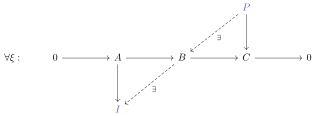Show that free implies projective using the universal properties, and conclude that every $$R{\hbox{-}}$$module has a projective cover.

Forming projective resolutions: take the minimal $$P_0 \xrightarrow[]{\delta_0} { \mathrel{\mkern-16mu}\rightarrow }\, M\to 0$$ such that $$\Omega^1 \mathrel{\vcenter{:}}=\ker \delta_0$$ has no projective summands. Continue in such a minimal way:For modules $$M$$ over an algebra $$A$$, if $$\dim_k(M)$$ is finite, then each $$P_i$$ can be chosen to be finite dimensional. Otherwise, define a complexity or rate of growth $$s c_A(M) \geq 0$$ such that $$\dim P_n \leq C n^{s-1}$$ for some constant $$C$$. A theorem we’ll prove is that $$s$$ is finite when $$A = k G$$ for every finite dimensional $$G{\hbox{-}}$$module. When $$A = kG$$, this is a numerical invariant but has a nice geometric interpretation in terms of support varieties $$V_A(M)$$, an affine algebraic variety where $$\dim V_A(M) = c_A(M)$$.

Recall the definition of a SES $$\xi: 0\to A \xrightarrow{d_1} B \xrightarrow{d_2} C\to 0$$ and show that TFAE:

• $$\xi$$ splits
• $$\xi$$ admits a right section $$s_r: C\to B$$
• $$\xi$$ admits a left section $$s_\ell B\to A$$

Hint: for the right section, show that $$s_r$$ is injective. Get that $$\operatorname{im}f + \operatorname{im}h \subseteq M_2$$, use exactness to write $$\operatorname{im}d_1 = \ker d_2$$ and show that $$\ker d_2 \cap\operatorname{im}s_r = \emptyset$$.

It’s not necessarily true that if $$B \cong A \oplus C$$ that $$\xi$$ splits: consider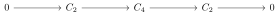Show that for $$P \in {\mathsf{R}{\hbox{-}}\mathsf{Mod}}$$, TFAE:

• $$P$$ is projective.
• Every SES $$\xi: 0\to A\to B\to P \to 0$$ splits.
• There exists a free module $$F$$ such that $$F = P \oplus K$$.

Show that $$\bigoplus_{i\in I} P_i$$ is projective iff each $$P_i$$ is projective.

• If $$R=k\in \mathsf{Field}$$, then every $$M\in {\mathsf{k}{\hbox{-}}\mathsf{Mod}}$$ is free and thus projective since $$M \cong \bigoplus_{i\in I} k$$ with $$k$$ free in $${\mathsf{k}{\hbox{-}}\mathsf{Mod}}$$.

• If $$R={\mathbb{Z}}$$, let $$P\in {\mathsf{{\mathbb{Z}}}{\hbox{-}}\mathsf{Mod}}$$ be projective and $$F$$ free and consider $$0\to K\to F\to P\to 0$$. Since $$F\cong P \oplus K$$, $$P$$ is a submodule of $$F$$, making $$P$$ free since $${\mathbb{Z}}$$ is a PID. So projective implies free.

• Not every $$M\in {\mathsf{{\mathbb{Z}}}{\hbox{-}}\mathsf{Mod}}$$ is projective: take $$C_6\in {\mathsf{{\mathbb{Z}}}{\hbox{-}}\mathsf{Mod}}$$, then $$C_6 \cong C_2 \oplus C_3$$ so $$C_2, C_3$$ are projective in $${\mathsf{C_6}{\hbox{-}}\mathsf{Mod}}$$ but not free here.

Let $$Q\in{\mathsf{R}{\hbox{-}}\mathsf{Mod}}$$ and show TFAE:

• $$Q$$ is injective
• Every SES $$\xi: 0\to Q\to B\to C\to 0$$ splits.

Show that $$\prod_{i\in I}Q_i$$ is injective iff each $$Q_i$$ is injective. Note that one needs to use direct products instead of direct sums here.

The category $${\mathsf{R}{\hbox{-}}\mathsf{Mod}}$$ has enough injectives, i.e. for every $$M\in{\mathsf{R}{\hbox{-}}\mathsf{Mod}}$$ there is an injective $$Q$$ and a SES $$0\to M\hookrightarrow Q$$.

See Hungerford or Weibel. Prove it first for $$\mathsf{C} = {\mathsf{Z}{\hbox{-}}\mathsf{Mod}}$$. The idea now is to apply \begin{align*} F({-}) \mathrel{\vcenter{:}}=\mathop{\mathrm{Hom}}_{\mathbb{Z}}(R,{-}): ({{\mathbb{Z}}}, {{\mathbb{Z}}}){\hbox{-}}\mathsf{biMod} &\to ({R}, {{\mathbb{Z}}}){\hbox{-}}\mathsf{biMod} ,\end{align*} the left-exact contravariant hom. Using that $$R\in ({R}, {R}){\hbox{-}}\mathsf{biMod}\hookrightarrow({{\mathbb{Z}}}, {R}){\hbox{-}}\mathsf{biMod}$$, one can use the right action $$R$$ on itself to define a left action on $$\mathop{\mathrm{Hom}}_{\mathbb{Z}}(R, M)$$. Then check that

• $$f$$ is left exact
• $$f$$ sends injectives to injectives.
• If $$R\in{\mathsf{{\mathbb{Z}}}{\hbox{-}}\mathsf{Mod}}$$ has an $$R{\hbox{-}}$$module structure, then $$F(R)$$ is again an $$R{\hbox{-}}$$module.

Show that for $$M\in{\mathsf{R}{\hbox{-}}\mathsf{Mod}}$$ that $$\mathop{\mathrm{Hom}}_{\mathbb{Z}}(R, M) \cong M$$.

Hint: try $$f\mapsto f(1)$$.

Next week:

• Tensor products
• Categories
• Tensor and Hom

# 3 Tensor Products (Tuesday, January 18)

Setup: $$R\in \mathsf{Ring}, M_R \in \mathsf{Mod}{\hbox{-}}\mathsf{R}$$, and $${}_R N \in \mathsf{R}{\hbox{-}}\mathsf{Mod}$$. Note that $$R$$ is not necessarily commutative. The goal is to define $$M\otimes_R N$$ as an abelian group.

The balanced product of $$M$$ and $$N$$ is a $$P \in {\mathsf{Ab}}{\mathsf{Grp}}$$ with a map $$f: M\times N\to P$$ such that

• $$f(x+x', y) = f(x, y) + f(x', y)$$
• $$f(x, y+y') = f(x,y) + f(x, y')$$
• $$f(ax, y) = f(x, ay)$$.

The tensor product $$(M\otimes_R N, \otimes)$$ of $$M$$ and $$N$$ is the initial balanced product, i.e. if $$P$$ is a balanced product with $$M\times N \xrightarrow{f} P$$ then there is a unique map $$\psi: M\otimes_R N\to P$$:Uniqueness follows from the standard argument on universal properties: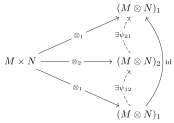Existence: let $$\mathsf{Free}({-}): {\mathsf{Set}}\to {\mathsf{Ab}}{\mathsf{Grp}}$$ and $$F\mathrel{\vcenter{:}}=\mathsf{Free}(M\times N)$$, then set $$M\otimes_R N \mathrel{\vcenter{:}}= F/G$$ where $$G$$ is generated by

• $$(x+x', y) - \qty{ (x, y) + (x', y) }$$
• $$(x, y+y') - \qty{ (x, y) + (x, y') }$$
• $$(ax, y) - (x, ay)$$.

Then define the map as \begin{align*} \otimes: M\times N\to F \\ (x, y) &\mapsto x\otimes y \mathrel{\vcenter{:}}=(x, y) + G .\end{align*}

Why it satisfies the universal property: use the universal property of free groups to get a map to $$F$$ and check that the following diagram commutes:Morphisms: for $$f:M\to M'$$ and $$g: N\to N'$$, form \begin{align*} f\otimes g: M\otimes N &\to M'\otimes N' \\ x\otimes y &\mapsto f(x) \otimes g(y) .\end{align*}

Note every $$z\in M\otimes_R N$$ is a simple tensor of the form $$z=x\otimes y$$!

• For $$R=k\in \mathsf{Field}$$, $$M\otimes_k N \in ({k}, {k}){\hbox{-}}\mathsf{biMod}$$. If $$M = \left\langle{m_i}\right\rangle$$ and $$N = \left\langle{n_j}\right\rangle$$, then $$M\otimes_k n = \left\langle{m_i\otimes n_j}\right\rangle$$ and $$\dim_k M\otimes_k N = \dim_k M \cdot \dim_k N$$.

• For $$A\in {\mathsf{Ab}}{\mathsf{Grp}}$$, $$A\otimes_{\mathbb{Z}}{\mathbb{Z}}\cong A$$ since $$x\otimes y = xy\otimes 1$$.

• $$M\mathrel{\vcenter{:}}= C_p\otimes_{\mathbb{Z}}{\mathbb{Q}}= 0$$. It suffices to check on simple tensors: \begin{align*} x\otimes y &= x\otimes{p\over p} y \\ &= x\otimes p\qty{1\over p} y \\ &= px\otimes\qty{1\over p} y \\ &= 0\otimes{1\over p}y \\ &= 0 .\end{align*}

• More generally, if $$A\in {\mathsf{Ab}}{\mathsf{Grp}}$$ is torsion then $$A\otimes_{\mathbb{Z}}{\mathbb{Q}}= 0$$.

A category $$\mathsf{C}$$ is a class of objects $$A\in \mathsf{C}$$ and for any pair $$(A, B)$$, a set of morphism $$\mathop{\mathrm{Hom}}_{\mathsf{C}}(A, B)$$ such that

1. $$(A, B) \neq (C, D)\implies \mathop{\mathrm{Hom}}(A, B)$$ and $$\mathop{\mathrm{Hom}}(C, D)$$ are disjoint.
2. Associativity of composition: $$(h\circ g)\circ f = h\circ(g\circ f)$$
3. Identities: $$\exists ! \operatorname{id}_A \in \mathop{\mathrm{Hom}}_{\mathsf{C}}(A, A)$$ for all $$A\in \mathsf{C}$$.

A subcategory $$\mathsf{D} \leq \mathsf{C}$$ is a subclass of objects and morphisms, and is full if $$\mathop{\mathrm{Hom}}_{\mathsf{D}}(A, B) = \mathop{\mathrm{Hom}}_{\mathsf{C}}(A, B)$$ for all objects in $$\mathsf{D}$$.

Examples of categories:

• $$\mathsf{C} = {\mathsf{Set}}$$,
• $$\mathsf{C} = {\mathsf{Grp}}$$,
• $$\mathsf{C} = {\mathsf{R}{\hbox{-}}\mathsf{Mod}}$$,
• $$\mathsf{C} = {\mathsf{Top}}$$ with continuous maps.

Examples of fullness:

• $${\mathsf{Grp}}\leq {\mathsf{Set}}$$ is not a full subcategory, since not all set morphisms are group morphisms.
• $${\mathsf{Ab}}{\mathsf{Grp}}\leq {\mathsf{Grp}}$$ is a full subcategory.

Recall the definition of covariant and contravariant functors, which requires that $$F(\operatorname{id}_A) = \operatorname{id}_{F(A)}$$.

# 4 Thursday, January 20

RIP Brian Parshall and Fred Cohen… 😔

Recall the definition of a covariant functor. Some examples:

• $$F(R) = U(R) = R^{\times}= {\mathbb{G}}_m(R)$$, the group of units of $$R$$.
• The forgetful functor $${\mathsf{Grp}}\to {\mathsf{Set}}$$.
• $$\mathop{\mathrm{Hom}}_{\mathbb{Z}}(R, {-})$$ for $$R\in ({{\mathbb{Z}}}, {R}){\hbox{-}}\mathsf{biMod}$$ is a functor $$\mathsf{{\mathbb{Z}}}{\hbox{-}}\mathsf{Mod}\to \mathsf{R}{\hbox{-}}\mathsf{Mod}$$.

Formulate $$\mathop{\mathrm{Hom}}_{\mathbb{Z}}({-}, {-})$$ in terms of functors between bimodule categories. How does this “use up an action” in the way $${-}\otimes_{\mathbb{Z}}{-}$$ does?

Recall that contravariant functors reverse arrows. Functors with the same variance can be composed.

Let $$F: \mathsf{C}\to \mathsf{D}$$ and consider the set map \begin{align*} F_{AB}: \mathop{\mathrm{Hom}}(A, B) &\to \mathop{\mathrm{Hom}}(FA, FB) \\ f &\mapsto F(f) .\end{align*} We say $$F$$ is full if $$F_{AB}$$ is injective for all $$A, B\in \mathsf{C}$$, and faithful if $$F_{AB}$$ is surjective for all $$A, B$$.

A morphism of functors $$\eta: F\to G$$ for $$F,G:\mathsf{C}\to \mathsf{D}$$ is a natural transformation: a family of maps $$\eta_A\in \mathop{\mathrm{Hom}}_{\mathsf{D}}(FA, GA)$$ satisfying the following naturality condition:If $$\eta_A$$ is an isomorphism for all $$A\in \mathsf{C}$$, then $$\eta$$ is a natural isomorphism.

For $$\mathsf{C}, \mathsf{D} = { \mathsf{Vect} }^{{\mathrm{fd}}}_{/ {k}}$$ finite-dimensional vector spaces, take $$F = \operatorname{id}$$ and $$G({-}) = ({-}) {}^{ \vee } {}^{ \vee }$$. Note that $$\mathop{\mathrm{Hom}}(FV, GV) \cong \mathop{\mathrm{Hom}}(V, V {}^{ \vee } {}^{ \vee }) \cong \mathop{\mathrm{Hom}}(V, V)$$, so set $$\eta_V$$ to be the image of $$\operatorname{id}_V$$ under this chain of isomorphisms. Show that $$\left\{{\eta_V }\right\}_{V\in \mathsf{C}}$$ assemble to a natural transformation $$F\to G$$.

Two categories $$\mathsf{C}, \mathsf{D}$$ are isomorphic if there are functors $$F, G$$ with $$F\circ G = \operatorname{id}_{\mathsf{D}}, G\circ F = \operatorname{id}_{\mathsf{C}}$$ equal to the identities. They are equivalent if $$F\circ G, G\circ F$$ are instead naturally isomorphic to the identity.

Some examples:

• $$\mathsf{C} = {\mathsf{Ab}}{\mathsf{Grp}}$$ and $$\mathsf{D} = {\mathsf{{\mathbb{Z}}}{\hbox{-}}\mathsf{Mod}}$$ by taking $$G:\mathsf{D}\to \mathsf{C}$$ the forgetful functor, and for $$F$$, using the same underlying set and defining the $${\mathbb{Z}}{\hbox{-}}$$module structure by $$n\cdot m \mathrel{\vcenter{:}}= m + m + \cdots + m$$.

• $$\mathsf{C}={\mathsf{R}{\hbox{-}}\mathsf{Mod}}$$ and $$\mathsf{D} = {\mathsf{\operatorname{Mat}_{n\times n}(R)}{\hbox{-}}\mathsf{Mod}}$$. For $${\mathsf{k}{\hbox{-}}\mathsf{Mod}}$$, the simple objects are $$k$$, but for $${\mathsf{\operatorname{Mat}_{n\times n}(R)}{\hbox{-}}\mathsf{Mod}}$$, the simple objects are $$k^n$$, so these categories are not isomorphic. However, it turns out that they are equivalent.

Producing inverse functors can be difficult, so we have the following:

Let $$F:\mathsf{C}\to \mathsf{D}$$, then there exists an inverse inducing an equivalence iff

• $$F$$ is fully faithful,
• Surjectivity on objects: for every $$A'\in \mathsf{D}$$, there exists an $$A\in \mathsf{C}$$ such that $$F(A) \cong A'$$.

$$\implies$$: Suppose $$F, G$$ induce an equivalence $$\mathsf{C} \simeq\mathsf{D}$$, so $$F\circ G\simeq\operatorname{id}_{\mathsf{D}}$$ and $$G\circ F \simeq\operatorname{id}_{\mathsf{C}}$$. To show $$f\to F(f)$$ is injective, check that \begin{align*} F(f) &= F(g) \\ \implies GF(f) &= GF(g) \\ \operatorname{id}(f) &= \operatorname{id}(g) \\ \implies f= g .\end{align*}

Show surjectivity.

A hint:

Let $$A'\in \mathsf{D}$$ with $$FG \simeq\operatorname{id}_{\mathsf{D}}$$ and $$\eta_{A'} \in \mathop{\mathrm{Hom}}_{\mathsf{D}}(A', FGA')$$ is an iso. Set $$A \mathrel{\vcenter{:}}= GA'\in \mathsf{C}$$ and use that \begin{align*} \mathop{\mathrm{Hom}}_{\mathsf{D}}(A', FGA') \mathrel{\vcenter{:}}=\mathop{\mathrm{Hom}}(A', FA) ,\end{align*} So if there is an isomorphism in $$\mathop{\mathrm{Hom}}(A', FA)$$, there exists an isomorphism in $$\mathop{\mathrm{Hom}}(FA, A')$$ and thus $$FA \cong A'$$.

#todo Missed a bit here so this doesn’t make sense as-is!

Let $$R\in \mathsf{Ring}$$ and set $$S\mathrel{\vcenter{:}}=\operatorname{Mat}_{n\times n}(R)$$, then $${\mathsf{R}{\hbox{-}}\mathsf{Mod}} \simeq{\mathsf{S}{\hbox{-}}\mathsf{Mod}}$$.

# 5 Tuesday, January 25

Recall isomorphisms $$\mathsf{C} \cong \mathsf{D}$$ of categories, so $$F\circ G = \operatorname{id}$$, vs equivalences of categories $$\mathsf{C} \simeq\mathsf{D}$$ so $$F\circ G \cong \operatorname{id}$$.

For $$F:\mathsf{C} \to \mathsf{D}$$ and $$G:\mathsf{D}\to \mathsf{C}$$ and write $$\psi_F: \mathop{\mathrm{Hom}}_{\mathsf{C}}(A, B) \to \mathop{\mathrm{Hom}}_{\mathsf{D}}(F(A), F(B))$$. This pair induces an equivalence iff

1. $$F$$ is faithful, i.e. $$\psi_F$$ is injective,
2. $$F$$ is full, i.e. $$\psi_F$$ is surjective,
3. For any $$D\in \mathsf{D}$$, there exists a $$C\in \mathsf{C}$$ with $$F(C) \cong D$$.

Let $$R\in \mathsf{Ring}$$ and $$S=\operatorname{Mat}_{n\times n}(R)$$, then $${\mathsf{R}{\hbox{-}}\mathsf{Mod}} \simeq{\mathsf{S}{\hbox{-}}\mathsf{Mod}}$$.

Define a functor $$F:{\mathsf{R}{\hbox{-}}\mathsf{Mod}} \to {\mathsf{S}{\hbox{-}}\mathsf{Mod}}$$ by $$F(M) \mathrel{\vcenter{:}}=\prod_{k\leq n} M$$, regarding this as a column vector and letting $$S$$ act by matrix multiplication. On morphisms, define $$F(f)(\mathbf{x}) = {\left[ {f(x_1), \cdots, f(x_n)} \right]}$$ for $$\mathbf{x} \in \prod M$$. Then $$F(\operatorname{id}) = \operatorname{id}$$, and (exercise) $$F(f)$$ is a morphism of $$S{\hbox{-}}$$modules and composes correctly: \begin{align*} F(g\circ f)(\mathbf{x}) = {\left[ {gf(x_1), \cdots, gf(x_n)} \right]} = F(g){\left[ {f(x_1), \cdots, f(x_n) } \right]} = \qty{ F(g)\circ F(f) } \mathbf{x} .\end{align*} So this defines a functor.

$$F$$ is fully faithful.

• Faithfulness: if $$F(f_1) = F(f_2)$$, then $$f_1(x_j) = f_2(x_j)$$ for all $$j$$, making $$f_1=f_2$$.

• Fullness: let $$g\in \mathop{\mathrm{Hom}}_S(M^n, N^n)$$ for $$M, N \in {\mathsf{R}{\hbox{-}}\mathsf{Mod}}$$ and $$e_{ij}$$ be the elementary matrix with a 1 only in the $$i, j$$ position. Check that $$e_{11} M^n = \left\{{{\left[ {x,0,\cdots} \right]} {~\mathrel{\Big\vert}~}x\in M}\right\}$$, $$e_{11} N^n = \left\{{{\left[ {y,0,\cdots} \right]}{~\mathrel{\Big\vert}~}y\in N}\right\}$$, and $$\operatorname{diag}(x)$$ be a matrix with only copies of $$x$$ on the diagonal. Then $$g(e_{11} M^n) \subseteq e_{11} g(M^n) \subseteq e_{11}N^n$$ and $$g{\left[ {x, 0, \cdots} \right]} = {\left[ {y, 0, \cdots} \right]}$$. Define $$f:M\to N$$ by $$f(x) = y$$, then on one hand, \begin{align*} g(\operatorname{diag}(a) {\left[ {x, 0,\cdots} \right]}) = g{\left[ {ax, 0, \cdots} \right]} = {\left[ {f(ax), 0, \cdots} \right]} ,\end{align*} but since $$g$$ is a morphism of $$S{\hbox{-}}$$modules, this also equals $$\operatorname{diag}(a)\cdot g{\left[ {x,0,\cdots} \right]} = {\left[ {ay,0,\cdots} \right]}$$. Then $$f(ax) = ay = af(x)$$, so $$f$$ is a morphism of $$R{\hbox{-}}$$modules.

Note that $$e_{j1} \mathbf{x} = {\left[ {0, \cdots, x,\cdots 0} \right]}$$ with $$x$$ in the $$j$$th position. Check that $$g(e_{j1}\mathbf{x}) = g{\left[ {0, \cdots, x, \cdots, 0} \right]}$$. The LHS is \begin{align*} e_{j1} g(\mathbf{x}) = e_{j1}{\left[ {f(x), 0, \cdots} \right]} = {\left[ { 0,\cdots, f(x), \cdots, 0} \right]} \end{align*} with $$f(x)$$ in the $$j$$th position. Hence $$g(\mathbf{x}) = {\left[ {f(x_1), \cdots, f(x_n)} \right]}$$, making $$F$$ full.

Show that \begin{align*} \qty{ \bigoplus _{\alpha \in I} M_\alpha } \otimes_R N &\cong \bigoplus _{\alpha\in I} \qty{M_\alpha \otimes_R N} ,\end{align*} and similarly for $$M\otimes(\oplus N_\alpha)$$.

Define functors $$F,G{\mathsf{R}{\hbox{-}}\mathsf{Mod}} \to{\mathsf{{\mathbb{Z}}}{\hbox{-}}\mathsf{Mod}}$$ by $$F({-}) \mathrel{\vcenter{:}}= M\otimes_R ({-})$$ and $$G({-}) \mathrel{\vcenter{:}}=({-})\otimes_R N$$ on objects, and on morphisms $$f:N\to N'$$, set $$F(f) \mathrel{\vcenter{:}}=\operatorname{id}\otimes f$$ and similarly for $$G$$. Recall the definition of exactness, left-exactness, and right-exactness.

Consider \begin{align*} \xi: 0\to p{\mathbb{Z}}\xrightarrow{f} {\mathbb{Z}}\xrightarrow{g} {\mathbb{Z}}/p{\mathbb{Z}}\to 0 \end{align*} and apply $$({-})\otimes_{\mathbb{Z}}{\mathbb{Z}}/p{\mathbb{Z}}$$. Use that $$p{\mathbb{Z}}\cong {\mathbb{Z}}$$ in $${\mathsf{{\mathbb{Z}}}{\hbox{-}}\mathsf{Mod}}$$ to get \begin{align*} F(\xi): C_p \xrightarrow{f\otimes\operatorname{id}} C_p \xrightarrow{g\otimes\operatorname{id}} C_p ,\end{align*} and \begin{align*} (f\otimes\operatorname{id})(px\otimes y) = px\otimes y = x\otimes py = 0 ,\end{align*} using that $$f$$ is the inclusion.

Show that $$M\otimes_R({-})$$ and $$({-})\otimes_R N$$ are right exact for any $$M, N \in {\mathsf{R}{\hbox{-}}\mathsf{Mod}}$$.

Let $$0 \to A \xrightarrow{f} B \xrightarrow{g} C \to 0$$ which maps to $$M\otimes A \xrightarrow{\operatorname{id}\otimes f} M\otimes B \xrightarrow{\operatorname{id}\otimes g} C$$.

• Show $$\operatorname{id}\otimes g$$ is surjective: write $$m\in M\otimes C$$ as $$m=\sum x_i\otimes y_j$$, pull back the $$y_j$$ via $$g$$ to get $$z_j$$ with $$g(z_j) = y_j$$. Then \begin{align*} (\operatorname{id}\otimes g)(\sum x_i \otimes z_J) = \sum x_i\otimes g(z_j) = \sum x_i \otimes y_j .\end{align*}

• Exactness, $$\operatorname{im}(\operatorname{id}\otimes f) = \ker (\operatorname{id}\otimes g)$$: Use that $$gf=0$$ by exactness of the original sequence, and $$(\operatorname{id}\otimes g)\circ (\operatorname{id}\otimes f) = \operatorname{id}\otimes(g\circ f) = 0$$, so $$\operatorname{im}(\operatorname{id}\otimes f) \subseteq \ker(\operatorname{id}\otimes g)$$.

• For the reverse containment, use that $$\operatorname{id}\otimes g: M\otimes B\to M\otimes C$$ and define a map \begin{align*} \Gamma: {M\otimes B \over \operatorname{im}(\operatorname{id}\otimes f)} \to M\otimes C \\ m\otimes n + \operatorname{im}(\operatorname{id}\otimes f)&\mapsto m\otimes g(n) .\end{align*} Then $$\phi$$ is an isomorphism iff $$\operatorname{im}(\operatorname{id}\otimes f) = \ker (\operatorname{id}\otimes g)$$. Define \begin{align*} \Psi: M\times C &\to {M\otimes B\over \operatorname{im}(\operatorname{id}\otimes f)} \\ (x, y) &\mapsto x \otimes z + \operatorname{im}(\operatorname{id}\otimes f) ,\end{align*} where $$g(z) = y$$, so $$z$$ is a lift of $$y$$.

Why is this well-defined? Check $$g(z_1) = y = g(z_2)$$ implies $$z_1 -z_2\in \ker g = \operatorname{im}f$$, so write $$f(y) = z_1-z_2$$ for some $$y$$. Then $$x\otimes z_1 + \operatorname{im}f = x\otimes z_2 + \operatorname{im}f$$.

Why does this factor through the tensor product? Check that $$\Psi$$ is a balanced product, this yields $$\mkern 1.5mu\overline{\mkern-1.5mu\Psi\mkern-1.5mu}\mkern 1.5mu: M\otimes C\to {M\otimes B\over \operatorname{im}(\operatorname{id}\otimes f)}$$. Now check that $$\mkern 1.5mu\overline{\mkern-1.5mu\Psi\mkern-1.5mu}\mkern 1.5mu, \Gamma$$ are mutually inverse: \begin{align*} \Gamma\Psi(x\otimes y) &= \Gamma(x\otimes z + \operatorname{im}(\operatorname{id}\otimes f)) = x\otimes g(z) = x\otimes y \\ \Psi\Gamma(x\otimes z + \operatorname{im}(\operatorname{id}\otimes f)) &= (x\otimes g(z) ) = x\otimes z + \operatorname{im}f .\end{align*}

When is $$M\otimes_R ({-})$$ exact?

# 6 Thursday, January 27

Recall that $$M\in {\mathsf{R}{\hbox{-}}\mathsf{Mod}}$$ is flat iff for every $$N, N'$$ and $$f\in \mathop{\mathrm{Hom}}_{{\mathsf{R}{\hbox{-}}\mathsf{Mod}}}(N, N')$$, the induced map \begin{align*} \operatorname{id}_M\otimes f: M\otimes_R N \to M\otimes_R N' \end{align*} is a monomorphism. Equivalently, $$M\otimes_R ({-})$$ is left exact and thus exact.

$$M \mathrel{\vcenter{:}}=\bigoplus _{\alpha\in I} M_\alpha$$ is flat iff $$M_\alpha$$ is flat for all $$\alpha\in I$$.

\begin{align*} M\otimes_R({-}) \mathrel{\vcenter{:}}=(\bigoplus M_\alpha)\otimes_R ({-}) \cong \bigoplus (M_\alpha \otimes_R ({-}) ) .\end{align*}

Show that projective $$\implies$$ flat.

Prove that the hom functors $$\mathop{\mathrm{Hom}}_{{\mathsf{R}{\hbox{-}}\mathsf{Mod}}}(M, {-}), \mathop{\mathrm{Hom}}_{{\mathsf{R}{\hbox{-}}\mathsf{Mod}}}({-}, M): {\mathsf{R}{\hbox{-}}\mathsf{Mod}}\to {\mathbb{Z}{\hbox{-}}\mathsf{Mod}}$$ are left exact.

Show that

• $$P$$ is projective iff $$\mathop{\mathrm{Hom}}_{{\mathsf{R}{\hbox{-}}\mathsf{Mod}}}(P, {-})$$ is exact
• $$I$$ is projective iff $$\mathop{\mathrm{Hom}}_{{\mathsf{R}{\hbox{-}}\mathsf{Mod}}}({-}, I)$$ is exact

An object $$Z\in \mathsf{C}$$ is a zero object iff $$\mathop{\mathrm{Hom}}_{\mathsf{C}}(A, Z), \mathop{\mathrm{Hom}}_{\mathsf{C}}(Z, A)$$ are singletons for all $$A\in \mathsf{C}$$. Write this as $$0_A \in \mathop{\mathrm{Hom}}_{\mathsf{C}}(A, Z)$$. If $$\mathsf{C}$$ has a zero object, define the zero morphism as $$0_{AB} \mathrel{\vcenter{:}}= 0_{B} \circ 0_A \in \mathop{\mathrm{Hom}}_{\mathsf{C}}(A, B)$$.

# 7 Tuesday, February 01

A category $$\mathsf{C}$$ is additive iff

• $$\mathsf{C}$$ has zero object
• There exists a binary operation $$+: \mathop{\mathrm{Hom}}(A, B){ {}^{ \scriptscriptstyle\times^{2} } }\to \mathop{\mathrm{Hom}}(A, B)$$ for all $$A, B\in \mathsf{C}$$ making $$\mathop{\mathrm{Hom}}(A ,B)$$ an abelian group.
• Distributivity with respect to composition: $$(g_1 + g_2)f = g_1f + g_2 f$$
• For any collection $$\left\{{A_1,\cdots, A_n}\right\}$$, there exists an object $$A$$, projections $$p_j: A\to A_j$$ with sections $$i_k: A_k\to A$$ with $$p_j i_j = \operatorname{id}_A$$, $$p_j i_k = 0$$ for $$j\neq k$$, and $$\sum i_j p_j = \operatorname{id}_A$$.

A morphism: $$k:K\to A$$ is monic iff whenever $$g_1, g_2: L\to K$$, $$kg_1 = kg_2 \implies g_1 = g_2$$: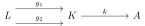Define $$k$$ to be epic by reversing the arrows.

Assume $$\mathsf{C}$$ has a zero object. Then for $$f:A\to B$$, the morphism $$k: K\to A$$ is the kernel of $$f$$ iff

• $$k$$ is monic
• $$fk=0$$
• For any $$g:G\to A$$ with $$fg=0$$, there exists a $$g'$$ with $$g=kg'$$.

For $$f\in {\mathsf{R}{\hbox{-}}\mathsf{Mod}}(A, B)$$, take $$k: \ker f\hookrightarrow A$$. If $$g\in \mathsf{C}(G, A)$$ with $$f(g(x)) = 0$$ for all $$x\in G$$, then $$\operatorname{im}g \subseteq \ker f$$ and we can factor $$g$$ as $$G \xrightarrow{g'} \ker f \xhookrightarrow{k} A$$.

For $$f: A\to B$$, a morphism $$c: B\to C$$ is a cokernel of $$f$$ iff

• $$c$$ is epic,
• $$cf=0$$
• For any $$h\in \mathsf{C}(B, H)$$ with $$hf=0$$, there is a lift $$h': C\to G$$ with $$h=h'c$$.

For $$\mathsf{C} = {\mathsf{R}{\hbox{-}}\mathsf{Mod}}$$ and $$f\in {\mathsf{R}{\hbox{-}}\mathsf{Mod}}(A, B)$$, set $$c: B\to B/\operatorname{im}f$$.

Show that kernels are unique. Sketch:

• Set $$k:K\to A$$, $$k': K'\to A$$.
• Factor $$k=k' u_1$$ and $$k' = ku_2$$.
• Then $$k\operatorname{id}= k(u_2 u_1) \implies \operatorname{id}= u_2 u_1$$, similarly $$u_1u_2=\operatorname{id}$$.

$$\mathsf{C}$$ is abelian iff $$\mathsf{C}$$ is additive and

• A5: Every morphism admits kernels and cokernels.
• A6: Every monic is the kernel of its cokernel, and every epic is the cokernel of its kernel.
• A7: Every morphism $$f$$ factors as $$f=me$$ with $$m$$ monic and $$e$$ epic.

For $$f\in {\mathsf{R}{\hbox{-}}\mathsf{Mod}}(A, B)$$,

• A5: Take $$k: \ker f\hookrightarrow A$$ and $$c: B\twoheadrightarrow B/\operatorname{im}f$$
• A6: For $$m: A\hookrightarrow B$$ monic, consider the composition $$A\hookrightarrow B \xrightarrow{\operatorname{coker}m} B/A$$ and check $$A\cong \ker(\operatorname{coker}m)$$.
• A7: Use the 1st isomorphism theorem:Some notes:

• Recall the definition the category of chain complexes $$\mathsf{Ch}(\mathsf{C})$$ over an abelian category: $$d_i d_{i+1} = 0$$, so $$\operatorname{im}d_i \subseteq \ker d_{i+1}$$.
• Every exact sequence is an acyclic complex.
• $$\mathsf{C}\hookrightarrow\mathsf{Ch}(\mathsf{C})$$ by $$M\mapsto \cdots \to 0 \to M \to 0 \to \cdots$$. Note that this isn’t an acyclic complex.
• Morphisms between complexes: chain maps, just levelwise maps forming commutative squares, i.e. maps commuting with the differentials.
• $$\mathsf{Ch}(\mathsf{C})$$ is additive: given $$\alpha_\bullet, \beta_\bullet\in \mathsf{Ch}\mathsf{C}( (A, d), (B, \delta) )$$, check that $$(\alpha_{i-1} + \beta_{i-1})d_i = \delta_i (\alpha_i + \beta_i)$$.
• There are direct sums: $$(A \oplus B)_i \mathrel{\vcenter{:}}= A_i \oplus B_i$$ with $$d \mathrel{\vcenter{:}}= d_A + d_B$$.
• Define cycles as $$Z_i \mathrel{\vcenter{:}}=\ker\qty{ C_i \xrightarrow{d_i} C_{i-1}}$$ for $$C_\bullet \in \mathsf{Ch}(\mathsf{C})$$, and boundaries $$B_i \mathrel{\vcenter{:}}=\operatorname{im}\qty{C_{i+1} \xrightarrow{d_{i+1}} C_i} \subseteq \ker d_i$$.
• Define $$H_i(C_\bullet )\mathrel{\vcenter{:}}= Z_i/B_i$$.
• Show that chain morphisms induce morphisms on homology:
• Let $$\alpha\in \mathsf{Ch}(\mathsf{C})(C, C')$$, then $$\alpha_i(Z_i) \subseteq Z_i'$$.
• Check $$d_2(a_i(Z_i)) = a_{i-1} d_i(Z_i) = 0$$.
• Factor $$Z_i \xrightarrow{\alpha_i} Z_i' \twoheadrightarrow Z_i'/B_i'$$.
• Show that $$x\in B_i$$ maps lands in $$B_i'$$ to get well-defined map on $$H_i$$.
• Use $$\alpha(B_i) \subseteq Z_i'$$, so pull back $$x\in B_i$$ to $$y\in C_{i+1}$$.
• Check $$d_{i+1}(y) = x$$, so $$\alpha(d_{i+1}(y)) = \alpha(x)$$.
• The LHS is $$d_{i+1}'(\alpha_{i+1}(y))$$, so $$\alpha_i(x) in \operatorname{im}d_{i+1}' = B_{i+1}'$$
• Chain homotopies: for $$\alpha, \beta\in \mathsf{Ch}(\mathsf{C})(C, C')$$, write $$\alpha \simeq\beta$$ iff there exists $$\left\{{s_i: C_i \to C_{i+1}' }\right\}$$ with $$\alpha_i - \beta_i = d_{i+1}' s_i + s_{i-1} d_i$$.# 8 Thursday, February 03

## 8.1 Projective Resolutions and Chain Maps

Also check that $$\simeq$$ is an equivalence relation, i.e. it is symmetric, transitive, and reflexive. For transitivity: given \begin{align*} \alpha_i - \beta_i &= d_{i+1}' s_i +s_{i-1} d_i \\ \beta_i - \gamma_i &= d_{i+1}' t_{i} + t_{i-1} d_i ,\end{align*} one can write \begin{align*} \alpha_i - \gamma_i &= d_{i+1}'(s_i + t_i) + (s_{i-1} + t_{i-1} ) d_i .\end{align*}

Let $$\alpha, \beta \in \mathsf{Ch}\mathsf{C}(A, B)$$ with induced maps $$\widehat{\alpha}, \widehat{\beta }\in \mathsf{Ch}\mathsf{C}(H^* A, H^* B)$$ on homology. If $$\alpha \simeq\beta$$, then $$\widehat{\alpha }= \widehat{\beta}$$.

A computation: \begin{align*} \widehat{\alpha}_{1}(&\left.z_{1}+B_{i}\right)=\alpha_{1}\left(z_{i}\right)+B_{i}^{\prime} \\ &=\beta_{i}\left(z_{i}\right)+\delta_{i+1}^{\prime} s_{1}\left(z_{i}\right)+s_{i-1}^{\prime \prime} \delta_{i}\left(z_{i}\right) + B_i'\\ &=\beta_{i}\left(z_{i}\right)+B_{i}^{\prime} \\ &=\widehat{\beta}_{i}\left(z_{i}+B_{i}\right) \end{align*}

• Homological algebra
• Commutative rings
• Support theory
• Tensor triangular geometry

Let $$M\in {\mathsf{R}{\hbox{-}}\mathsf{Mod}}$$. A projective complex for $$M$$ is a chain complex $$(C_i, d_i)_{i\in {\mathbb{Z}}}$$, indexed homologically: \begin{align*} \cdots \to C_2 \xrightarrow{d_2} C_1 \xrightarrow{d_1} C_0 \xrightarrow{d_0\mathrel{\vcenter{:}}={\varepsilon}} 0 .\end{align*}

In particular, $$d^2 = 0$$, but this complex need not be exact. A projective resolution of $$M$$ is an exact projective complex in the following sense:

• $$H_{k\geq 1}({ {C}_{\scriptscriptstyle \bullet}} ) = 0$$
• $$H_0({ {C}_{\scriptscriptstyle \bullet}} ) = C_0/d(C_1) = C_0/\ker {\varepsilon}\cong M$$.

Some projective resolutions:

• For $$M\in {\mathsf{R}{\hbox{-}}\mathsf{Mod}}$$, projective resolutions exist since we can find covers by free modules: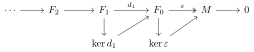• For $$M\in {\mathsf{Z}{\hbox{-}}\mathsf{Mod}}$$, every module has a 2-stage resolution: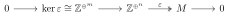For $$\mu \in \mathsf{C}(M, M')$$ and $$C \mathrel{\vcenter{:}}=({ {C}_{\scriptscriptstyle \bullet}} , d) \twoheadrightarrow M, C' \mathrel{\vcenter{:}}=({ {C}_{\scriptscriptstyle \bullet}} ', d')\twoheadrightarrow M'$$, there is an induced chain map $$\alpha \in \mathsf{Ch}\mathsf{C}(C, C')$$. Moreover, any other chain map $$\beta$$ is chain homotopic to $$\alpha$$.

Note that $$C$$ can in fact be any projective complex over $$M$$, not necessarily a resolution.

Using that $$C_0$$ is projective, there is a lift of the following form:Now inductively, we want to construct the following lift:STS $$\operatorname{im}\alpha_{n-1} d_n \subseteq \ker d_{n-1}'$$, which follows from \begin{align*} d_{n-1}' \alpha_{n-1} d_n(x) = \alpha_{n-1} d_{n-1} d_n(x) .\end{align*}

So there is a map $$C_n \to \operatorname{im}d_n'$$, and using projectivity produces the desired lift by the same argument as in the case case: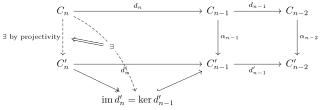To see that any two such maps are chain homotopic, set $$\gamma \mathrel{\vcenter{:}}=\alpha - \beta$$, then \begin{align*} {\varepsilon}'( \gamma_0) = {\varepsilon}'( \alpha_i - \beta_i) = \mu{\varepsilon}- \mu {\varepsilon}=0 ,\end{align*} and \begin{align*} d_n'(\gamma_n) &- d_n'( \alpha_n - \beta_n) \\ &= d_n' \alpha_n - d_n' \beta_n \\ &= \alpha_{n-1} d_n - \beta_{n-1} d_n \\ &= \gamma_{n-1} d_n ,\end{align*} so $$\gamma$$ yields a well-defined chain map.

We’ll now construct the chain homotopy inductively. There is a lift $$s_0$$ of the following form:This follows because $$\operatorname{im}d_1' = \ker {\varepsilon}'$$ and $${\varepsilon}' \gamma_0 = 0$$ by the previous calculation.

Assuming all $$s_{i\leq n-1}$$ are constructed, set $$\gamma_i = d_{i+1}' s_i + s_{i-1} d_i$$. Setting $$\gamma_n - s_{n-1}d_n: C_n \to C_n'$$, then \begin{align*} d_n'( \gamma_n - s_{n-1} d_n) &= d_n' \gamma_n - d_n' s_{n-1} d_n \\ &= \gamma_{n-1} d_n - d_n' s_{n-1} d_n \\ &= (\gamma_{n-1} - d_n' s_{n-1})d_n \\ &= s_{n-2} d_{n-1} d_n \\ &= 0 ,\end{align*} using $$d^2 = 0$$. Now there is a lift $$s_n$$ of the following form:Thus follows from the fact that $$\operatorname{im}\gamma_n - s_{n-1} d_n \subseteq \ker d_n'$$ and projectivity of $$C_n$$.

Dually one can construct injective resolutions $$0 \to M \xhookrightarrow{\eta} { {D}_{\scriptscriptstyle \bullet}}$$

## 8.2 Derived Functors

Setup: $$F: {\mathsf{R}{\hbox{-}}\mathsf{Mod}}\to {\mathbb{Z}{\hbox{-}}\mathsf{Mod}}$$ is an additive covariant functor, e.g. $$({-}) \otimes_R N$$ or $$M\otimes_R({-})$$, and $${ {C}_{\scriptscriptstyle \bullet}} \xrightarrow[]{{\varepsilon}} { \mathrel{\mkern-16mu}\rightarrow }\, M$$ a complex over $$M$$. We define the left-derived functors as $$(L_n F)(M) \mathrel{\vcenter{:}}= H_n(F({ {C}_{\scriptscriptstyle \bullet}} ))$$.

# 9 Tuesday, February 08

Defining derived functors: for $$F$$ an additive functor and $$M\in {\mathsf{R}{\hbox{-}}\mathsf{Mod}}$$, take a projective resolution and apply $$F$$: \begin{align*} \cdots \to C_2 \xrightarrow{d_2} C_1 \xrightarrow{d_1} C_0 \xrightarrow{{\varepsilon}= d_0} M \to 0 \leadsto F(C_2) \xrightarrow{Fd_2} F(C_1) \xrightarrow{Fd_1} \cdots ,\end{align*} so $${ {C}_{\scriptscriptstyle \bullet}} \rightrightarrows F$$.

Define the left-derived functor \begin{align*} {\mathbb{L}}F M \mathrel{\vcenter{:}}= H_n F{ {C}_{\scriptscriptstyle \bullet}} .\end{align*}

Any $$\mu \in {\mathsf{R}{\hbox{-}}\mathsf{Mod}}(M, M')$$ induces a chain map $$\widehat{\alpha }\in \mathsf{Ch}{\mathsf{R}{\hbox{-}}\mathsf{Mod}}(H_* F{ {C}_{\scriptscriptstyle \bullet}} , H_* F{ {C}_{\scriptscriptstyle \bullet}} ' )$$, where $$\alpha$$ is any lift of $$\mu$$ to their resolutions.Show that any two lifts $$\alpha, \alpha'$$ induce the same map on homology.

Similarly, $${\mathbb{L}}F(M)$$ does not depend on the choice of resolution:For $$0\to M' \to M\to M'' \to 0$$ in $$\mathsf{C}$$, a projective resolution is a collection of chain maps forming projective resolutions of each of the constituent modules:Show that such resolutions exist. This involves constructing $${\varepsilon}: C_0 \to M$$:The claim is that $${\varepsilon}(x, x'') \mathrel{\vcenter{:}}=\gamma {\varepsilon}'(x') + {\varepsilon}^*(x'')$$ works. To prove surjectivity, use the following:

Given a commutative diagram of the following formIf $$g,h$$ are mono (resp. epi, resp. iso) then $$f$$ is mono (resp. epi, resp. iso).

• Let $$x\in M$$

• Set $$y=\sigma(x)$$

• Find $$z\in C_0$$ such that $${\varepsilon}'' p_0 (z) = y$$.

• Consider $${\varepsilon}(z) - x$$ and apply $$\sigma$$: \begin{align*} \sigma({\varepsilon}(z) - x) &= \sigma {\varepsilon}(x) - \sigma(x) \\ &= {\varepsilon}'' p_0(x) - \sigma(x) \\ &= y-y \\ &= 0 .\end{align*}

• So $${\varepsilon}(z) - x\in \ker \sigma = \operatorname{im}\gamma$$

• Pull back to $$w\in C_0'$$ such that $$\gamma {\varepsilon}'(w) = {\varepsilon}(z) - x$$

• Check $${\varepsilon}i_0 (w) = \gamma {\varepsilon}'(w) = {\varepsilon}(z) - x$$, so $${\varepsilon}(i_0(w) - z) = -x$$.

The setup:This is exact and commutative by a diagram chase:

• $$f = i \circ \downarrow_{\ker {\varepsilon}'}$$ shows $$g(\ker {\varepsilon}) \subseteq \ker {\varepsilon}''$$
• $$g = p \circ \downarrow_{\ker {\varepsilon}}$$ shows $$f(\ker {\varepsilon}') \subseteq \ker {\varepsilon}$$.

To show exactness along the top line:

• $$f$$ is injective, since it’s the restriction of an injective map.
• $$g$$ is surjective:
• Let $$x\in \ker {\varepsilon}''$$, so $${\varepsilon}''(x) = 0$$.
• $$\exists y\in C_0$$ with $$p_0(y) = x$$ by surjectivity of $$p_0$$.
• Check $${\varepsilon}''(p_0(y)) = {\varepsilon}(x) = 0$$ in $$M''$$, so $$\sigma{\varepsilon}(y) = 0$$
• Thus $${\varepsilon}(y)\in \ker \sigma = \operatorname{im}\gamma$$
• By surjectivity there exists $$w \in C_0'$$ such that $$\gamma( {\varepsilon}'(w)) = {\varepsilon}(y)$$.
• Use commutativity to verify \begin{align*} {\varepsilon}(i_0(w) - y) &= {\varepsilon}(i_0(w)) - {\varepsilon}(y) \\ &= \gamma{\varepsilon}'(w) - {\varepsilon}(y) \\ &= {\varepsilon}(y) - {\varepsilon}(y) \\ &= 0 .\end{align*}
• Then \begin{align*} g(i_0(w) - y) &= p_0(i_0 (w)) - g(y) \\ &= -g(y) \\ &= -p_0(y) \\ &= -x .\end{align*}
• Exactness at the middle, i.e. $$\operatorname{im}f = \ker g$$:
• $$\operatorname{im}f \subseteq \ker g$$ by exactness of the second row, so it STS $$\ker g \subseteq \operatorname{im}f$$.
• Let $$y\in \ker g$$, then by commutativity $$y\in \ker p_0 = \operatorname{im}i_0$$. Note that $$y\in \ker {\varepsilon}$$ by definition.
• Write $$y = i_0(x)$$ for some $$x\in C_0'$$
• Note $$\gamma {\varepsilon}' (x) = {\varepsilon}i_0(x) = {\varepsilon}(y) = 0$$ since $$y\in \ker {\varepsilon}$$.
• Since $$\gamma'$$ is mono, $${\varepsilon}'(x) = 0$$, so $$y = i_0(x) = f(x)$$.

For $$F: {\mathsf{R}{\hbox{-}}\mathsf{Mod}}\to{\mathbb{Z}{\hbox{-}}\mathsf{Mod}}$$ additive and a SES \begin{align*} \xi: 0\to M' \xrightarrow{f} M \xrightarrow{g} M'' \to 0 ,\end{align*}

note that there are morphisms \begin{align*} {\mathbb{L}}F M'' \to {\mathbb{L}}F M\to {\mathbb{L}}FM' .\end{align*}

There is a connecting morphism \begin{align*} \Delta: {\mathbb{L}}F M'' \to \Sigma^{-1} {\mathbb{L}}F M' ,\end{align*} which in components looks like